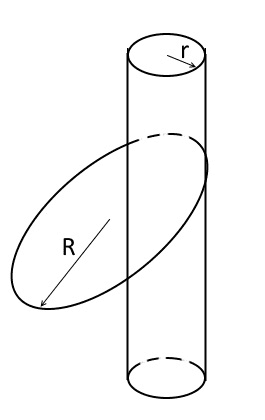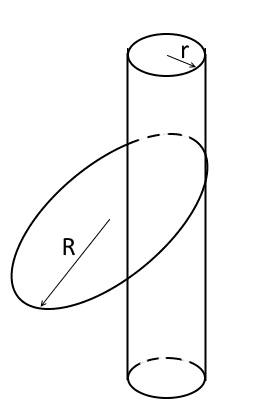# Hula-hoop problem

I would like to propose an olympic (as it seems to me) level problem.

There is a vertical pillar of radius r and a thin hoop of mass m and radius R( R>r).
The hoop putted on the pillar rotates such that the center of the hoop describes a circle in horizontal plan and the velocity of the center remains constant in its absolute value. The is no slipping between the hoop and the pillar. The hoop does not slides down being undergone with the gravity.
Show that if R>4r/3 then, for some suitable values of the velocity and the friction coefficient between the hoop and the pillar, the described above motion is possible. And if R<4r/3 then independently on the friction and the velocity such a motion does not exist.What do you think about the problem? I do not ask a solution :) I just expect teaching professional comments

berkeman
Mentor
I would like to propose an olympic (as it seems to me) level problem.

There is a vertical pillar of radius r and a thin hoop of mass m and radius R( R>r).
The hoop putted on the pillar rotates such that the center of the hoop describes a circle in horizontal plan and the velocity of the center remains constant in its absolute value. The is no slipping between the hoop and the pillar. The hoop does not slides down being undergone with the gravity.
Show that if R>4r/3 then, for some suitable values of the velocity and the friction coefficient between the hoop and the pillar, the described above motion is possible. And if R<4r/3 then independently on the friction and the velocity such a motion does not exist.What do you think about the problem? I do not ask a solution :) I just expect teaching professional comments
Welcome to the PF.

Are you a teacher asking about the difficulty level of this problem? Or are you a student looking for help on a schoolwork problem? We are trying to figure out which sub-forum here at the PF your thread fits into the best.Are you a teacher asking about the difficulty level of this problem?

yes I am

Last edited by a moderator:
mfb
Mentor
Did you solve it?

It is certainly beyond the typical homework problem. After thinking about the problem for 10 minutes, I don't see why there should be a minimal radius. The coefficient of friction and the angular velocity would have to be large, but it should work out.

Edit: Wait, found a mistake.
Edit2: Fixed, didn't change the conclusion.

I composed this problem for an olympiad in theoretical mechanics not for ordinary homework. To solve it one must write down the equations of the rigid body motion and look at them

mfb
Mentor
That's what I did.
Setting the cylinder radius to 1, and with ##\theta## as tilt of the hoop relative to the horizontal, I get ##\tan (\theta) \omega^2 (R \cos (\theta) - 1) = g## as condition for the angle. With sufficiently large ω this has a solution for all R>1. It will also satisfy the geometric boundary condition of ##R \cos (\theta) > 1##. The required coefficient of friction is ##\mu \geq \tan (\theta)##.

That's what I did.

#### Attachments

• hula-hoop-eng.pdf
36 KB · Views: 438
mfb
Mentor
I guess [a,b] means the cross product?
I think that rotating coordinate system makes everything more complicated than necessary. The hoops angular velocity in the S frame should be constant (it is always in the z direction with constant magnitude), and if you drop the acceleration term there the constraint on R disappears.

I guess [a,b] means the cross product?
yes

The hoops angular velocity in the S frame should be constant (it is always in the z direction with constant magnitude)
sure, this is exactly what was written. But the angular velocity vector rotates relative to the inertial frame. The equation of angular momentum is also written with respect to the inertial frame, this equation is just expanded in the basis $e_x,e_y,e_z$ .

mfb
Mentor
But the angular velocity vector rotates relative to the inertial frame.
That's what the other ##\omega## does, right?
Hmm, confusing.